# QlikView Documents

QlikView documentation and resources.

Announcements
Document boards are being consolidated, this board no longer allows NEW documents READ MORE

# Aggr() and set analysis: set modifiers inside and / or outside aggr()?!?

cancel
Showing results for
Did you mean:Anonymous
Not applicable

## Aggr() and set analysis: set modifiers inside and / or outside aggr()?!?

Hi community,

"Where should the set modifier be placed? Inside and/or outside the aggr()?"

"If you are unsure, you should place the set modifiers inside and outside the aggr(). This will probably lead to the desired result..."

I just can't agree. In my opinion it really, really! depends...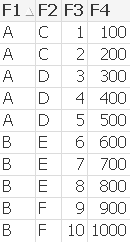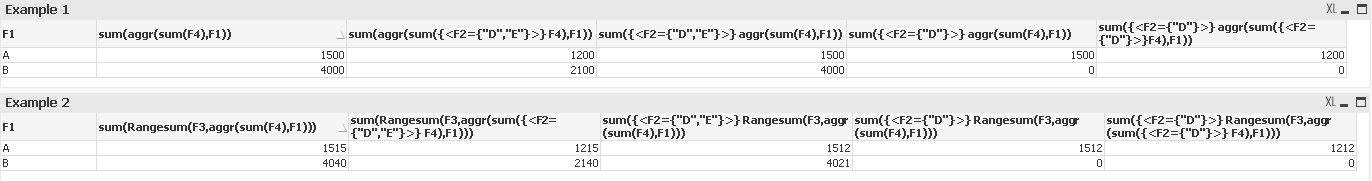Example 1

sum(aggr(sum(F4),F1))

--> default case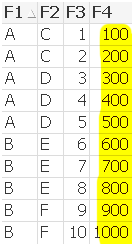sum(aggr(sum({<F2={"D","E"}>} F4),F1))

--> set modifier at inner aggregation limits F4 values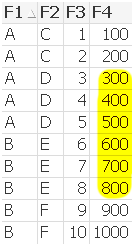sum({<F2={"D","E"}>} Rangesum(F3,aggr(sum(F4),F1)))

--> set modifier at outer aggregation

--> no difference , because F1={A} exists for F2={D} and F1={B} exists for D2={E}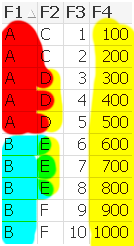sum({<F2={"D"}>} aggr(sum(F4),F1))

--> set modifier at outer aggregation

--> F1={A} exists for F2={D} and F1={B} doesn‘t exist for D2={E}sum({<F2={"D"}>} aggr(sum({<F2={"D"}>}F4),F1))

--> set modifier at inner and outer aggregation

--> F1={A} exists for F2={D} and F1={B} doesn‘t exist for D2={E}

--> set modifier at inner aggregation limits F4 values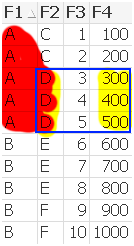Example 2

sum(Rangesum(F3,aggr(sum(F4),F1)))

--> default case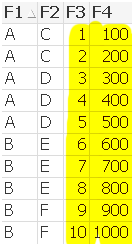sum(Rangesum(F3,aggr(sum({<F2={"D","E"}>} F4),F1)))

--> set modifier at inner aggregation limits F4 values inside aggr()

--> but doesn’t limit F3 outside aggr()sum({<F2={"D","E"}>} Rangesum(F3,aggr(sum(F4),F1)))

--> set modifier at outer aggregation limits only F3 field, because F1={A} exists for F2={D} and F1={B} exists for D2={E}sum({<F2={"D"}>} Rangesum(F3,aggr(sum(F4),F1)))

--> set modifier at outer aggregation

--> limits F3 and F4, because F1={A} exists for F2={D} and F1={B} doesn‘t exist for D2={E}sum({<F2={"D"}>} Rangesum(F3,aggr(sum({<F2={"D"}>} F4),F1)))

--> set modifier at inner and outer aggregation limts F3 and F4 values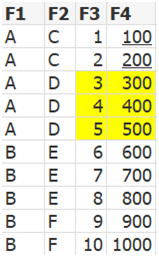Regards

Robin

Labels (3)

• ### New to QlikView